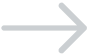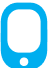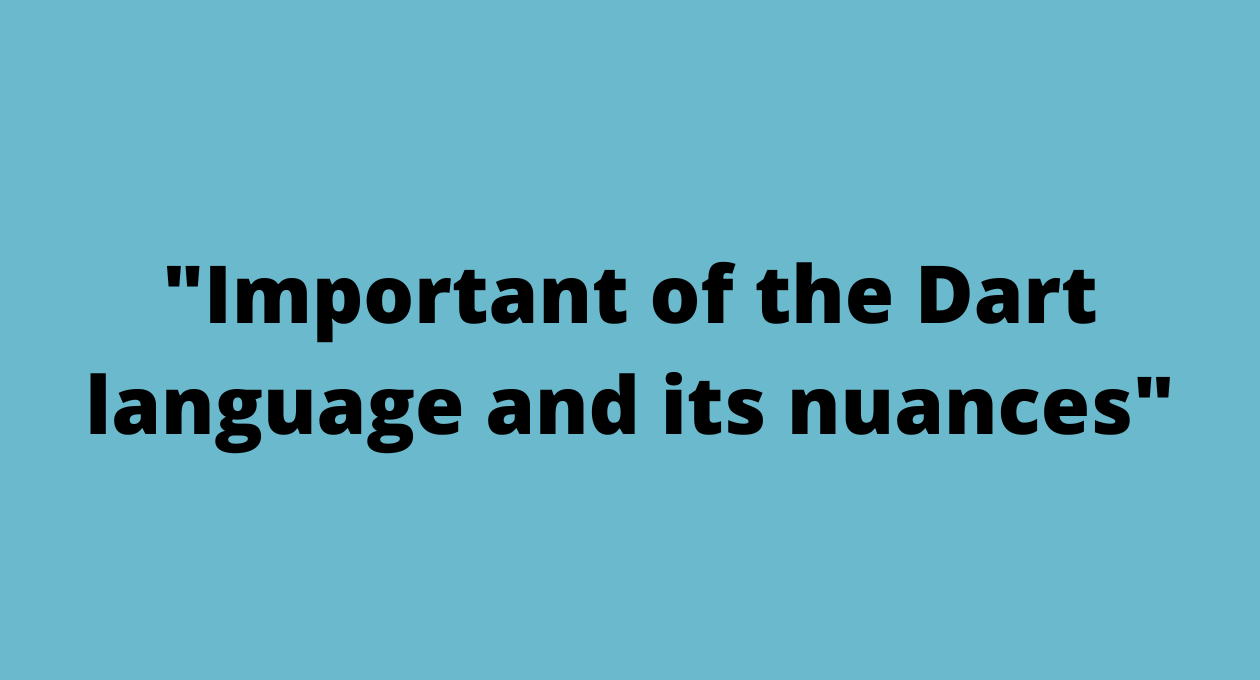• You can reach us through•+91-984-5825982
•+91-996-4689921
•sales@cumulations.com
•Whatsapp Us!

# Important of the Dart language and its nuances.###### Cumulations.

14 Jun 2022For a Flutter developer, it is important to understand the Dart language and its nuances.

Generally, when we want to start with Flutter, we skim through the Dart tutorials and may not spend enough time understanding the nitty-gritty of the language.

Here is the list of dart concepts that developers in a flutter app development company think are important to understand and also to keep looking at it every now and then to refresh/sharpen our dart basics.

We think it would be beneficial for Flutter developers to spend time analyzing these notes and also run the sample code available to better understand the concepts.

# Essential Dart notes

```String variables adjacent to each other get concatenated.
print ("hello" "hi"

"my name is Praveen"

);

}```
• `//hellohimy name is Praveen`
```“Var” variable gets resolved to its type based on the initial value and you can’t change it later.
var x=1;

x="ss";

print(x);

Object y=1;

y="ss";

print(y);

/*Error: A value of type 'String' can't be assigned to a variable of type 'int'.

x="ss"```
• `  */`
```Null value initialization is by default.
var x=null; //no need

x=10;```
• `  print(x);`
```Late variables are never initialized if you don't use them.
void main() {

late int h1=hello();

int x1=2;

print(x1);

//hello function is not called at all

}

int hello(){

print("you are in hello function");

return 2;

}```
• `// 2,`
```Final and Const: you cannot modify elements of a list if it is declared as const.
final list1={1,2,3,4};

print(list1);

const Map map={'key':"value"};

map["key"]="valu2";

print(map);```
• `//Uncaught Error: Unsupported operation: Cannot modify unmodifiable Map`
```Functions can have positional arguments and named arguments. Positional arguments can have default values when they are optional only.
int normalFunction(int one, [int addBy=0]) {

}

int namedFunction({var one=0, var inputs=1}){

return  inputs+3;

}

void main(){

print(namedFunction(inputs:1));

print(normalFunction(1));

```
• `}`
```All named arguments for functions are optional unless they are marked with keyword ‘required’.
helloFunctions({required String vehicle, String? owner, int? wheels}) {

owner ??=

""; // what does this do? initialize if the variable is not initialized

print("hello functions " + owner);

}

void main() {

print("hello");

helloFunctions(vehicle: "car");

• `}`
```Functions can be assigned to a variable and then can be called using that variable.

}

void main() {

final list1={1,2,3,4};

var a=f(2);

var b=f;

print(a);

print(b(4));

}```
• `//3 and 5`
```Default parameter values should be compile-time constants.
int normalFunction([List list=const [1,2,3,4]]) {

return list.length;

}

void main(){

print(normalFunction([1,2]));

}

```
• `//2`

```Functions can take another function as a parameter.
int normalFunction([List list=const [1,2,3,4]]) {

return list.length;

}

int functionTakingAnotherFunctionAsParam(Function f, [List arg=const [1,2]]){

return f(arg);

//here the function is actually called with () brackets

}

void main(){

print(functionTakingAnotherFunctionAsParam( normalFunction));

//while passing the function just the name is mentioned

}```
```Do you know this ~/ operator? Gives the integer output of the division
num x=12.5;

print(x/2);

print(x~/2);```
• `//6.25 6`
```Do you know what “IS” operator is all about? Is double variable an int
void main(){

int x=1;

double y=2.6;

print(x is double);

print(y is int);

animal a=animal();

monkey m=monkey();

print(a is monkey );

print(m is animal );

}

class animal{

}

class monkey extends animal{

}

```
• `//true false false true`
```Do you know what is “??” operator?
void main(){

num a=10;

num? b=null;

int x=123;

b ??= x;

a ??=x;

print(b);

print(a);

}```
• `//123 10`
```Every nonempty switch case should be broken ( or continue, break, rethrow).
var command = 'CLOSED';

switch (command) {

case 'CLOSED': // Empty case falls through.//no break is ok here

case 'NOW_CLOSED':

// Runs for both CLOSED and NOW_CLOSED.

executeNowClosed();

break;  //error here  if there is no break```
• `}`
```Constructors of the classes are functions of the same name as of class or with the name following pattern <class-name>.<identifier>
class Point {

int? x;

int? y;

Point.fromMap(Map<String,int> maps){

this.x=maps["x"];

this.y=maps["y"];

}

toString(){

return this.x.toString()+ this.y.toString();

}

}

void main(){

Map<String,int> m={"x":10,"y":12};

print(Point.fromMap(m));

```
• `}`
```No constructor overloading? You can have only one unnamed constructor.
class A{

A(){

}

A(int a){

}

}

```
• `//Error: unnamed constructor is already defined`
```The default constructor is available only when you don't have any other constructor in the class.
class Point {

int? x;

Point.namedConstrcutor(int x){

this.x=x;

}

}

void main(){

Point p=new Point(); //cant do this

}```
• `//error`
```Initializer List: list of items the constructor can take and use for initializing the instance variables.
/* here we are using the initializer list to initialize the final variable

which otherwise would  have to do it in the declaration line only */

class Shape{

final int? finalVariablex;

Shape.namedConstructor(argumentx): finalVariablexx=argumentx{

print("Inside the Shape's named constructor");

}

}

//you can also initialize a final variable if you use the syntactic sugar method ```
• `//Shape(this.finalVariablex)`
```Constructors are not inherited by the subclass and they have to be called explicitly if the parent class doesn't have a default (no-argument) constructor.
class Rectangle{

double l=0;

double b=0;

Rectangle(len,bre){

l=len;

b=bre;

print("rectangle constructor");

}

}

class Square extends Rectangle{

//if you don't call super here it throws error

Square(len):super(len,len){

print("square constructor");

}

}

void main(){

Square r=Square(10);

}

//rectangle constructor```
• `//square constructor`
```The order of execution when creating the object of subclass: 1. Initiilizerlist 2, parent class constructor 3. Derived class constructor
void main() {

Square s=Square(10);

}

class Shape{

Shape.namedConstructor() {

print("Inside the Shape's named constructor");

}

}

class Rectangle extends Shape{

double length=0;

static sprint(b){

return b;

}

print("Inside the Rectangle's constructor");

}

//super constructor call has to be the last in the initializers list

}

class Square extends Rectangle{

static staticFunction(x){

print("inside function call of Square");

return x;

}

Square(len):super(staticFunction(len),len){

print("Inside the Square' constructor");

}```
• `}`

Factory Constructors are the constructor methods that may not create a brand new object each time. Can be used for use cases involving singleton creation and usage.

```class Square {

final  double? len;

static Square _sq=new Square._getDeafult(10);

Square._getDeafult(this.len)  { }

factory Square.getSquare(){

return _sq;

}

}

void main(){

Square r=Square.getSquare();

Square r2=Square.getSquare();

print(r.len);

print(r2==r);

```
• `}`

A class can be implemented as an interface. Each class that implements a class interface should provide methods for each of the variables with its getter and setter methods.

```class Person{

String? secondVariable;

String? name;

greet(){

print(name);

}

}

class NewPerson implements Person{

@override

String? secondVariable;

String? _tempName;

NewPerson(this._tempName);

String? get name =>   _tempName; // if you make it name it will result in stackoverflow

set name (String? x) => _tempName=x;

greet(){

print(_tempName);

}

}

void main(){

Person  n = NewPerson("sss");

n.greet(); ```
• `}`

Can one constructor pass the buck to another? Sure, only if takes its hands-off completely

```class Point {

double x, y;

// The main constructor for this class.

Point():x=0,y=0;

Point.newPoint(double x1, double x2): this._alongXAxis(x1);

// Delegates to the main constructor.

Point._alongXAxis(double x) : this();

//{}because the redirecting constructor cannot have the body

}

void main (){

/* the function is accessible because

In Dart, the privacy is at library level rather than class level

*/

Point p3=Point._alongXAxis(10);

Point p=Point.newPoint(1,5);

```
• `}`
```Use the spread operator to initialize another list item. You can use for and if conditions while creating the list.
void main() {

List list1 = [1, 2, 4, 6];

List list2 = [0, ...list1];```
• `}`
```Do you think the list can store items of different types?
void main() {

List l = ["Aa", 1, 2, 1.4, "332"];

print(l);```
• `}`

Can you initialize an empty “set” using the “var” keyword?
void main() {

// var setVariable = {}; This would have been MAP

var setVariable = <int>{};

var mapVariable = <String, int>{};

mapVariable[“He”] = 1;

print(setVariable);

print(mapVariable);

• }
```In Dart, exceptions are all unchecked meaning you don't have to declare the function with the potential exception type it might throw.
//no throws declaration or anything

//exceptions unchecked

exceptionFunc() {

print("exception functions");

throw 3;

}

void main() {

print("hello");

exceptionFunc();```
• `}`
```In Dart, you can throw any object not just the objects of subtype of exception and error type.
exceptionFunc() {

print("exception functions");

throw 3; //throwing the

}

void main() {

print("hello");

exceptionFunc();```
• `}`
```Enums are sealed meaning they cannot be initiated, instantiated, subclassed or implemented.
enum Vehicle{

car, bike,bus;

}

```
• `// Vehicle v= Vehicle();`
```Enhanced enums: where the custom classes can be enums.
enum color {

blue,

black,

white;

}

enum Vehicle {

bike(2, "naveen");

final int wheels;

final String owner;

const Vehicle(this.wheels, this.owner);

}

void main() {

print(Vehicle.car.wheels);

print("owner is " + Vehicle.car.owner);

print("name is " + Vehicle.car.name); //check how this is coming

//if you remove the generic type usage

//for Vehicle it would not know that name is present

List<Vehicle> l = Vehicle.values;

l.forEach((Vehicle element) {

print((element.index).toString());

print(element.name);

});```
• `}`
```Enums indexing starts from 0 and you can use values to get the list of enums and name.
enum Vehicle {```

car,

bike,

bus;

}

void main() {

List<Vehicle> l = Vehicle.values;

//values provide you the list of enums

l.forEach((element) {

print(“” + element.index.toString() + ” ” + element.name);

//if you remove Vehicle from List’s generic  List<Vehicle> annotation you cant access name variable

});

• }
```Enums can be accessed just like static class members.
enum Vehicle {

car,

bike,

bus;

}

void main() {

Vehicle car = Vehicle.car;

print(car.name);

print(car.index);```
• `}`
```Mixin allows us to extend the ability to reuse the code.
mixin Person {

String? name;

}

class Vehicle {

int? wheels;

String? type;

int? speed;

Vehicle(this.wheels, this.type, this.speed);

}

//with is the keyword

class Car extends Vehicle with Person {

Car(int speed, String dname) : super(4, "Car", speed) {

this.name = dname;

}

String? get driver => name;

}

void main() {

Car benz = Car(130, "Pra");

print(benz.driver);

Car audi = Car(170, "BSB");

print(audi.driver);```
• `}`
```How to make a function return a future? make it async and then the return type would be a future.
void helloFun() {```

print(“Normal functions”);

}

Future<void> futurehelloFun() async {

print(“Future functions”);

• }
```“Await for” loop have you heard of? If you want to get events from the stream until the stream ends ( unless you break or return from the for loop)
await for (final i in serv){```
• `}`
```If you want to receive events for “Stream”? then you have to LISTEN!
//this is a generator function```

//check the sign async* here

Stream<int> naturalNumber() async* {

int i = 0;

while (i < 10) {

yield i; //you can use yield* and call the same function recursively

i++;

}

}

void main() async {

print(“hello”);

// await for (int s in naturalNumber()) {

//   print(s);

// }

naturalNumber()

.listen((event) => print(event), onDone: () => print(“completed”));

//listen function accepts one positional arguments and rest of optional named arguments

• }
```How to extend a class with the new methods without modifying the class?
class Hello {}

extension extendedMethod on Hello {

printer() {

print("hello");

}

}

void main() {

Hello h = new Hello();

h.printer();

var c = h;

// this is where the dart's type inference is working

c.printer();

dynamic d=h;

// not possible here d.printer()```
• `}`
```Type inference of Dart? What is an example?
void main() {

var l = {1: 1, 1: "s"};

var mal = {1: 1, 12: 2};

l.putIfAbsent(3, () => "s");

mal.putIfAbsent(3, () => "s"); //error wh6=y? because var determines the type

//  change it to   var mal = <int, String>{1: 1, 12: 2}; it will work

print(l);```
• `}`
```Isolate: How to listen in the main isolate?
import 'dart:io';

import 'dart:isolate';

// generator function which will simply generate a stream

Stream<dynamic> sendingName() async* {

for (int i = 0; i < 10; i++) {

yield "Praveen" + i.toString();

}

}

createIsolateAndWait(SendPort p) async {

print("Inside a new isolate");

sendingName().listen((event) {

p.send(event);

}, onDone: () {

print("Isolate finished its job");

p.send(null);

Isolate.exit();

});

}

void main() async {

print("calling an isolate");

Isolate.spawn(createIsolateAndWait, p.sendPort);

//var p = sendingName();

p.listen((message) {

if (message == null)

p.close(); // not sure why I am being forced here to close.

else

print("got" + message);

}, onDone: () => print("main isolate is done now"));```
• `}`
```What is the difference between forEach and for in?
void main() {

List l = [1, 3, 5, 6];

l.forEach((element) {

print(element);

});

for (int i in l) {

print(i);```
• `  }`
```Which is better? tempList.elementAt or tempList?
void main() {

List l = [1, 3, 5, 6];

//In Dart, an Iterable is an abstract class,

// List implements this class and hence the methods because of iterable are also accessible.

print(l +

"" +

l.elementAt(

3)); // its method in iterable and it always goes sequentially and hence

//not an optimal option```
• `}`